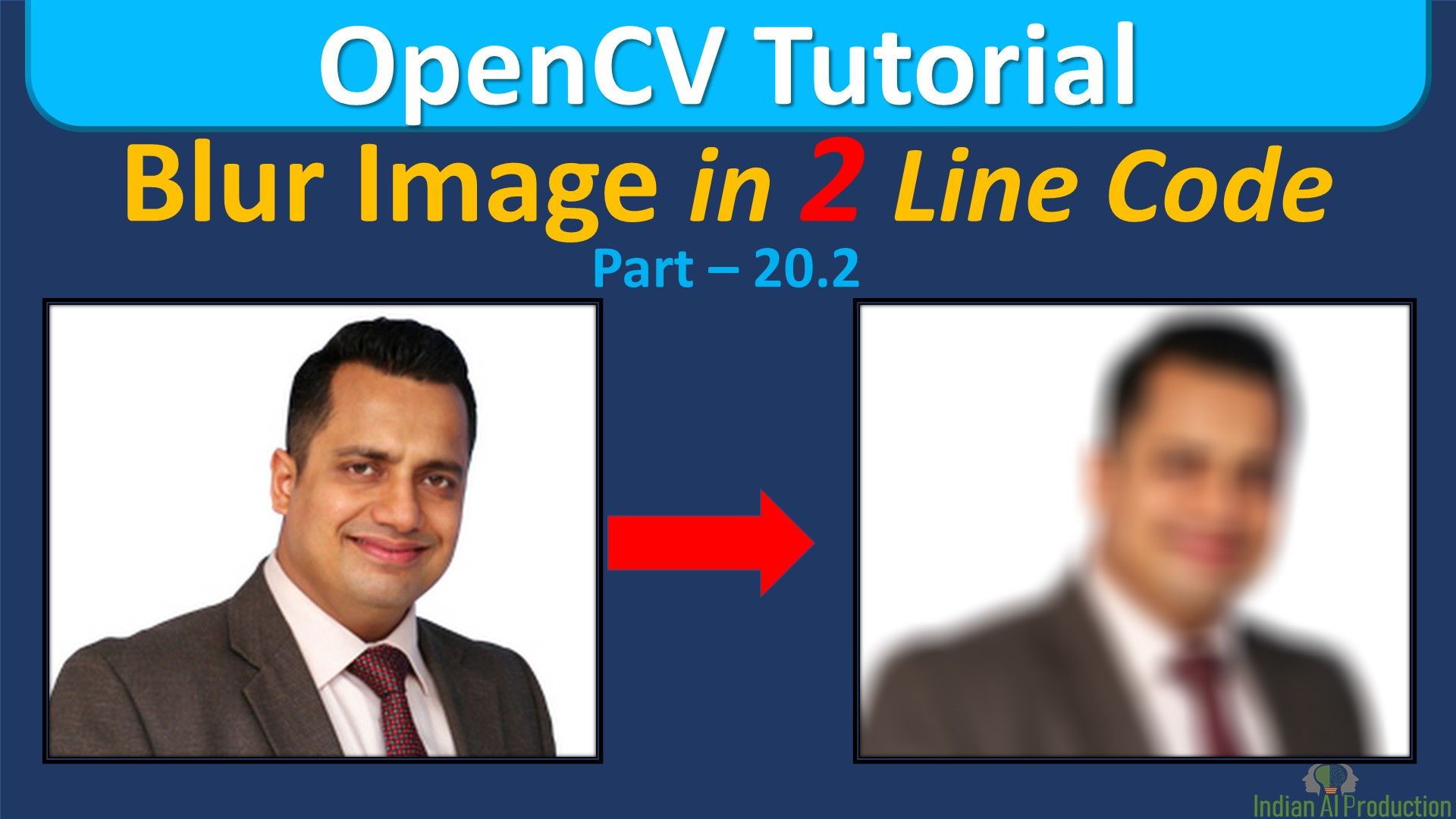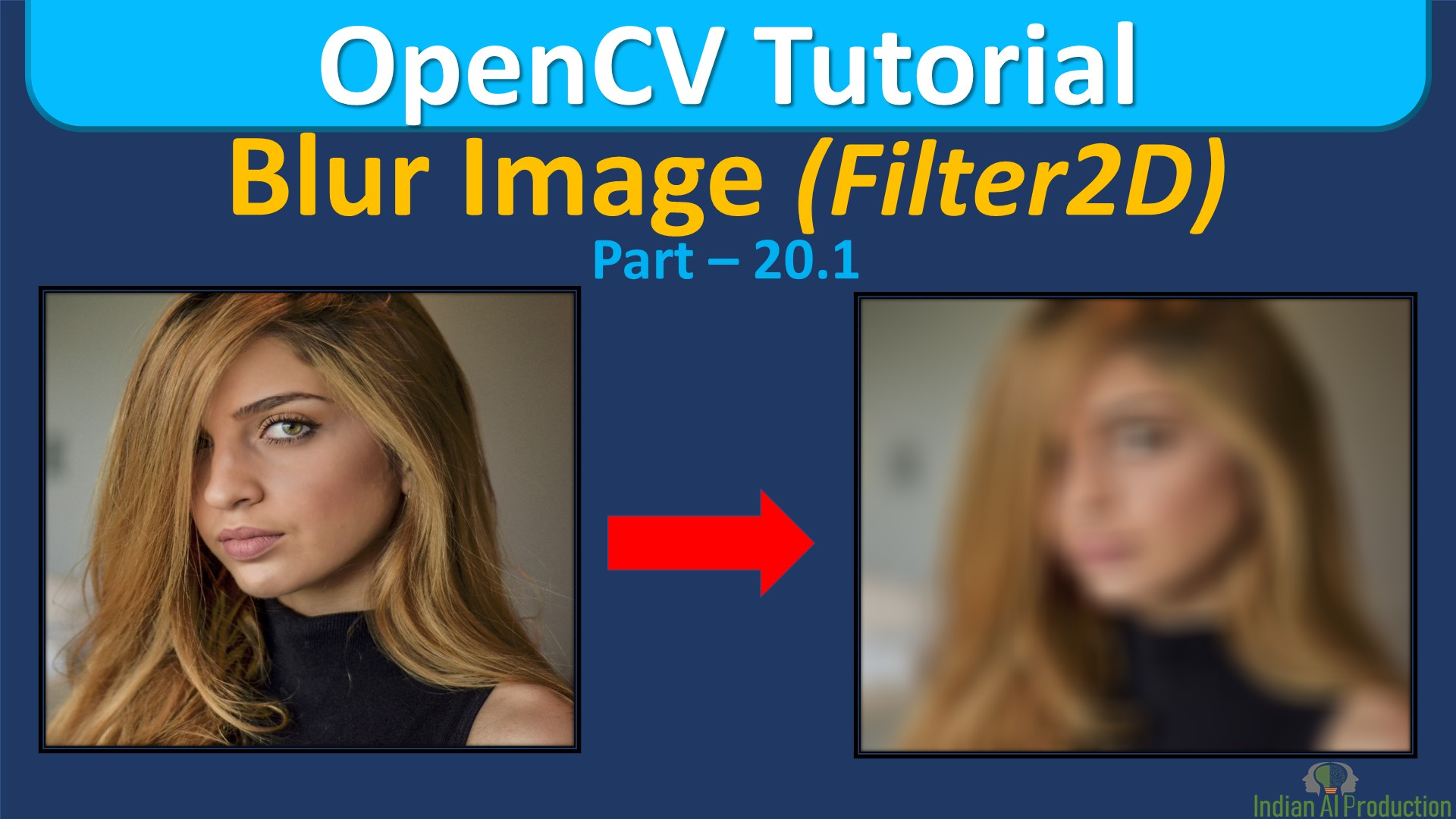## Blur Image Using cv2.blur() & cv2.boxFilter()OpenCV Python | OpenCV Tutorial

In Python OpenCV Tutorial, Explained How to Blur image using cv2.blur() and cv2.boxFilter() opencv function. Get the answers of below questions: How do I blur an image in OpenCV?How do you blur an image in Python?Why do we blur image?How do you blur part of a picture? Syntax: cv2.blur(src, ksize ]]) -> dst @brief Blurs an image using the normalized box filter. Parameters:...## Blur Image using filter2d OpenCV Python | OpenCV Tutorial

In Python OpenCV Tutorial, Explained How to Blur image using cv2.filter2d() opencv function. Get the answers of below questions: How do I blur an image in OpenCV?How do you blur an image in Python?Why do we blur image?How do you blur part of a picture? Syntax: cv2.filter2D(src, ddepth, kernel ]]]) -> dst Parameters: . @param src input image. . @param dst output image of the same size and the...
Top